# Engineering Mechanics - Force Vectors

### Exercise :: Force Vectors - General Questions

• Force Vectors - General Questions
6.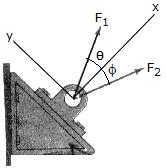If F1 = F2 = 30lb, determine the anglesand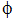so that the resultant force is directed along the positive x axis and has a magnitude of FR = 20 lb.

 A.== 70.5° B.== 41.4° C.== 19.47° D.== 18.43°

Explanation:

No answer description available for this question. Let us discuss.

7.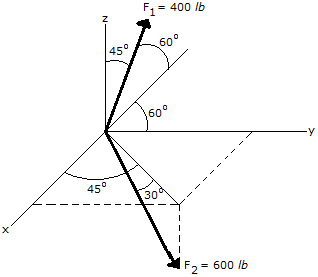Express the Force F2 in Cartesian vector form.

 A. F2 = (155 i + 155 j + 300 k) lb B. F2 = (212 i + 212 j - 519 k) lb C. F2 = (155 i + 155 j - 300 k) lb D. F2 = (367 i + 367 j - 300 k) lb

Explanation:

No answer description available for this question. Let us discuss.

8.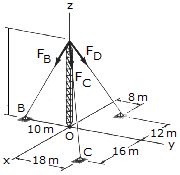The antenna tower is supported by three cables. The forces in these cables are as follows: FB = 520 N, FC = 680 N, and FD = 560 N. Write the resultant of these three forces as a vector.

 A. R = (4i +16j-72k) N B. R = (-120i +40 j-960k) N C. R = (560i +720j+1440k) N D. R = (80i +320j-1440k) N

Explanation:

No answer description available for this question. Let us discuss.

9.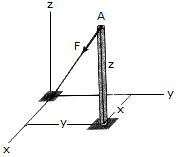The cable AO exerts a force on the top of the pole of F = {—120i — 90j — 80k} lb. If the cable has a length of 34 ft, determine the height z of the pole and the location (x,y) of its base.

 A. x = 16 ft, y = 16 ft, z = 25 ft B. x = 12 ft, y = 9 ft, z = 8 ft C. x = 20 ft, y = 10 ft, z = 14 ft D. x = 24 ft, y = 18 ft, z = 16 ft

Explanation:

No answer description available for this question. Let us discuss.

10.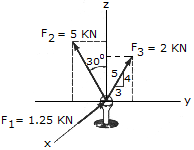The ball joint is subjected to the three forces shown. Find the magnitude of the resultant force.

 A. R = 5.30 kN B. R = 5.74 kN C. R = 5.03 kN D. R = 6.20 kN